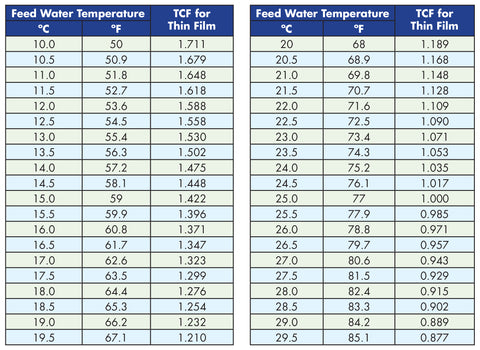# Temperature Correct Flow

The equation is Rated Permeate Flow ÷ TCF (Temperature Correction Factor from Table below) = Temperature Correct Flow

For example:

If you want to produce 150 GPD and the source water temperature is 59° the Temperature Correct Flow equation is 150 ÷ 1.422 = 105 GPD.

The approximate amount of water produced per day would be 105. In order to achieve the desired 150 GPD than you would have to go with a system that is rated more than 150 GPD.